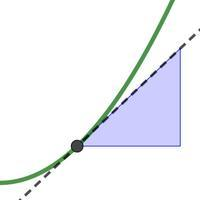# Introducing DerivativesDifferentiation allows to find rates of change. The derivative is the rate at which one quantity changes with respect to an other. When we differentiate a function we find the gradient function. On a graph, the derivative is the slope of...

To access the contents of this site, you need to log in or subscribe